# Search Results (36)

View
Selected filters:
• Exponents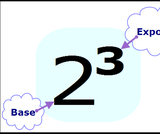Conditions of Use:
Remix and Share
Rating

Students understand the meaning of exponents.

Subject:
Mathematics
Material Type:
Lesson Plan
Author:
Angela Vanderbloom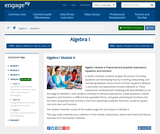Conditions of Use:
Remix and Share
Rating

In earlier modules, students analyze the process of solving equations and developing fluency in writing, interpreting, and translating between various forms of linear equations (Module 1) and linear and exponential functions (Module 3). These experiences combined with modeling with data (Module 2), set the stage for Module 4. Here students continue to interpret expressions, create equations, rewrite equations and functions in different but equivalent forms, and graph and interpret functions, but this time using polynomial functions, and more specifically quadratic functions, as well as square root and cube root functions.

Subject:
Algebra
Material Type:
Module
Provider:
New York State Education Department
Provider Set:
EngageNY
09/17/2013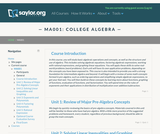Conditions of Use:
Rating

This course is also intended to provide the student with a strong foundation for intermediate algebra and beyond. Upon successful completion of this course, you will be able to: simplify and solve linear equations and expressions including problems with absolute values and applications; solve linear inequalities; find equations of lines; and solve application problems; add, subtract, multiply, and divide various types of polynomials; factor polynomials, and simplify square roots; evaluate, simplify, multiply, divide, add, and subtract rational expressions, and solve basic applications of rational expressions. This free course may be completed online at any time. It has been developed through a partnership with the Washington State Board for Community and Technical Colleges; the Saylor Foundation has modified some WSBCTC materials. (Mathematics 001)

Subject:
Algebra
Material Type:
Full Course
Provider:
The Saylor Foundation
04/16/2012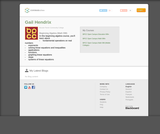Rating

In this beginning algebra course, you'll learn about
fundamental operations on real numbers
exponents
solving linear equations and inequalities
applications
functions
graphing linear equations
slope
systems of linear equations

Subject:
Algebra
Material Type:
Full Course
Lecture
Lesson
Module
Provider:
Bossier Parish Community College
Author:
Gail Hendrix
10/16/2017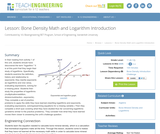Conditions of Use:
Rating

In their reading from activity 1 of this unit, students should have discovered the term "logarithm." It is at this point that they begin their study of logarithms. Specifically, students examine the definition, history and relationship to exponents; they rewrite exponents as logarithms and vice versa, evaluating expressions, solving for a missing piece. Students then study the properties of logarithms (multiplication/addition, division/subtraction, exponents). They complete a set of practice problems to apply the skills they have learned (rewriting logarithms and exponents, evaluating expressions, solving/examining equations for a missing variable.) Then they complete a short quiz covering what they have studied thus far concerning logarithms (problems similar to the practice problems). They consider how what they have learned moves them closer to answering the unit's challenge question.

Subject:
Engineering
Material Type:
Lesson Plan
Provider:
TeachEngineering
Provider Set:
TeachEngineering
Author:
Kristyn Shaffer
09/18/2014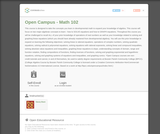Rating

This course is designed to take the concepts you learn in developmental math to expand your knowledge of algebra. This course will focus on two major algebraic concepts to learn - how to SOLVE equations and how to GRAPH equations. Throughout this course you will be challenged to recall ALL of your prior knowledge of operations of real numbers as well as your knowledge related to solving and graphing linear equations (which you should have already mastered from developmental algebra). You will use this prior knowledge to expand on learning the following objectives: solving linear & rational equations. operations of complex numbers, solving quadratic equations, solving radical & polynomial equations, solving equations with rational exponents, solving linear and compound inequalities, solving absolute value equations and inequalities, graphing linear equations & slope, understanding concepts of domain, range and function notation, finding compositions of functions, finding inverses of functions, solving and graphing exponential and logarithmic equations, solving and graphing systems of equations and inequalities, and graphing conics.

*Open Campus courses are non-credit tutorials and cannot, in and of themselves, be used to satisfy degree requirements at Bossier Parish Community College (BPCC). (College Algebra Course by Bossier Parish Community College is licensed under a Creative Commons Attribution-NonCommercial-NoDerivatives 4.0 International License. Based on a work at http://bpcc.edu/opencampus/index.html.)

Subject:
Mathematics
Algebra
Material Type:
Full Course
Provider:
Bossier Parish Community College
Author:
Stacey Black
10/11/2017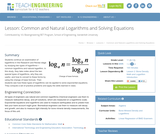Conditions of Use:
Rating

Students continue an examination of logarithms in the Research and Revise stage by studying two types of logarithms—common logarithms and natural logarithm. In this study, they take notes about the two special types of logarithms, why they are useful, and how to convert to these forms by using the change of base formula. Then students see how these types of logarithms can be applied to solve exponential equations. They compute a set of practice problems and apply the skills learned in class.

Subject:
Engineering
Material Type:
Lesson Plan
Provider:
TeachEngineering
Provider Set:
TeachEngineering
Author:
Kristyn Shaffer
09/18/2014Conditions of Use:
No Strings Attached
Rating

The purpose of this task is to introduce the idea of exponential growth and then connect that growth to expressions involving exponents. It illustrates well how fast exponential expressions grow.

Subject:
Mathematics
Material Type:
Activity/Lab
Provider:
Illustrative Mathematics
Provider Set:
Illustrative Mathematics
Author:
Illustrative Mathematics
05/01/2012Rating

This math problem demonstrates the concept of geometric progression, through an example of a million dollar contract between an employee and an employer. Application of the concept of geometric progression to social cause activism is addressed. This resource is from PUMAS - Practical Uses of Math and Science - a collection of brief examples created by scientists and engineers showing how math and science topics taught in K-12 classes have real world applications.

Subject:
Mathematics
Material Type:
Lecture Notes
Provider:
NASA
Provider Set:
NASA Wavelength
11/05/2014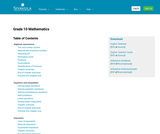Rating

This is a comprehensive math textbook for Grade 10. It can be downloaded, read on-line on a mobile phone, computer or iPad. Every chapter has links to on-line video lessons and explanations. Summary presentations at the end of each chapter offer an overview of the content covered, with key points highlighted for easy revision. Topics covered are: algebraic expressions, equations and inequalities, exponents, number patterns, functions, finance and growth, trigonometry, analytical geometry, statistics, probability, Euclidean geometry, measurements. This book is based upon the original Free High School Science Text series.

Subject:
Mathematics
Material Type:
Textbook
Provider:
Siyavula
04/12/2012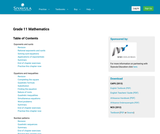Rating

Subject:
Mathematics
Material Type:
Textbook
Provider:
Siyavula
04/12/2012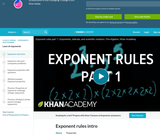Conditions of Use:
Remix and Share
Rating

This 10-minute video lesson looks at exponent rules.

Subject:
Algebra
Material Type:
Lecture
Provider:
Provider Set:
Author:
Salman Khan
10/11/2012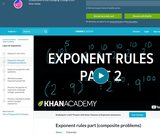Conditions of Use:
Remix and Share
Rating

This 8-minute video lesson looks at exponent rules.

Subject:
Algebra
Material Type:
Lecture
Provider:
Provider Set:
Author:
Salman Khan
10/11/2012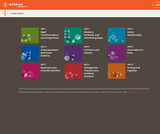Conditions of Use:
No Strings Attached
Rating

Student-facing 8th grade math resources. Covers transformations & congruence, slope, similarity, linear relationships, associations in data, volume, functions, scientific notation, the pythagorean theorem and irrational numbers.

Subject:
Mathematics
Material Type:
Unit of Study
Provider:
Open Up Resources
05/21/2019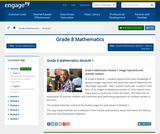Conditions of Use:
Remix and Share
Rating

In Grade 8 Module 1, students expand their basic knowledge of positive integer exponents and prove the Laws of Exponents for any integer exponent.  Next, students work with numbers in the form of an integer multiplied by a power of 10 to express how many times as much one is than the other.  This leads into an explanation of scientific notation and continued work performing operations on numbers written in this form.

Subject:
Numbers and Operations
Material Type:
Module
Provider:
New York State Education Department
Provider Set:
EngageNY
05/14/2013Conditions of Use:
Remix and Share
Rating

In this classic hands-on activity, learners estimate the length of a molecule by floating a fatty acid (oleic acid) on water. This lab asks learners to record measurements and make calculations related to volume, diameter, area, and height. Learners also convert meters into nanometers. Includes teacher and student worksheets but lacks in depth procedure information. The author suggests educators search the web for more complete lab instructions.

Subject:
Chemistry
Material Type:
Activity/Lab
Provider:
Exploratorium
Author:
Eric Muller
The Exploratorium
11/07/2007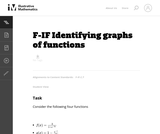Conditions of Use:
No Strings Attached
Rating

The goal of this task is to get students to focus on the shape of the graph of the equation y=ex and how this changes depending on the sign of the exponent and on whether the exponential is in the numerator or denominator. It is also intended to develop familiarity, in the case of f and k, with the functions which are used in logistic growth models, further examined in ``Logistic Growth Model, Explicit Case'' and ``Logistic Growth Model, Abstract Verson.''

Subject:
Mathematics
Functions
Material Type:
Activity/Lab
Provider:
Illustrative Mathematics
Provider Set:
Illustrative Mathematics
Author:
Illustrative Mathematics
08/17/2012Rating

This lesson unit is intended to help you assess how well students are able to: Perform arithmetic operations, including those involving whole-number exponents, recognizing and applying the conventional order of operations; Write and evaluate numerical expressions from diagrammatic representations and be able to identify equivalent expressions; apply the distributive and commutative properties appropriately; and use the method for finding areas of compound rectangles.

Subject:
Geometry
Material Type:
Assessment
Lesson Plan
Provider:
Shell Center for Mathematical Education
U.C. Berkeley
Provider Set:
Mathematics Assessment Project (MAP)
Mathematics Assessment Project (MAP)
04/26/2013Conditions of Use:
Remix and Share
Rating

Subject:
Mathematics
Material Type:
Full Course
Provider:
Pearson
10/06/2016Conditions of Use:
Remix and Share
Rating

Expressions

Type of Unit: Concept

Prior Knowledge

Students should be able to:

Write and evaluate simple expressions that record calculations with numbers.
Use parentheses, brackets, or braces in numerical expressions and evaluate expressions with these symbols.
Interpret numerical expressions without evaluating them.

Lesson Flow

Students learn to write and evaluate numerical expressions involving the four basic arithmetic operations and whole-number exponents. In specific contexts, they create and interpret numerical expressions and evaluate them. Then students move on to algebraic expressions, in which letters stand for numbers. In specific contexts, students simplify algebraic expressions and evaluate them for given values of the variables. Students learn about and use the vocabulary of algebraic expressions. Then they identify equivalent expressions and apply properties of operations, such as the distributive property, to generate equivalent expressions. Finally, students use geometric models to explore greatest common factors and least common multiples.

Subject:
Mathematics
Algebra
Material Type:
Unit of Study
Provider:
Pearson